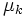# Coulomb model of friction

View other constitutive relations

## Definition

The Coulomb model of friction, or the Amonton-Coulomb model of friction, or Coulomb friction, refers to a simple model of dry friction that is fairly accurate empirically. Specifically, it is a model that helps predict the direction and magnitude of the friction force between two bodies with dry surfaces in contact.

For more on how to apply this model of friction to mechanics scenarios, see applying the Coulomb model of friction.

## Model for static friction

Further information: Static friction

Static friction occurs when there is no slipping between the two surfaces of contact. This can occur both when the two bodies are in rest relative to each other, and when the surfaces are rolling against each other.

There exists a constant$\mu_s$, termed the limiting coefficient of static friction, dependent on the nature of the two surfaces in contact, such that the static friction is determined by the following rules:

• Static friction is equal in magnitude and opposite in direction to the net external force acting along the plane of contact that is causing a tendency for the surfaces to slip against each other. In other words, it acts to precisely cancel out the external force that would otherwise have caused slipping, thus producing no slipping.
• The maximum value of static friction that can be experienced through the surface of contact equals$\mu_s N$, where$\mu_s$ is the limiting coefficient of static friction and$N$ is the normal force. In the limiting case, the two surfaces are said to just start slipping against each other.

The proportionality with normal force, and the fact that this constant of proportionality is dependent on the nature of the surfaces in contact, is termed Amonton's second law of friction. The fact that the magnitude of area of contact plays no role is termed Amonton's first law of friction.

## Model for kinetic friction

Further information: Kinetic friction

Kinetic friction, or dynamic friction, occurs when there is slipping between the two surfaces of contact. There exists a constant$\mu_k$, termed the coefficient of kinetic friction, dependent on the nature of the two surfaces in contact, such that, for sufficiently small speeds of slipping:

• Kinetic friction is opposite in direction to the direction of actual slipping. Its direction does not depend on the direction of other external forces.
• The magnitude of kinetic friction is$\mu_kN$, where$\mu_k$ is the coefficient of kinetic friction, and$N$ is the normal force between the bodies.$\mu_k$ for a given pair of surfaces is typically somewhat less than$\mu_s$, indicating that once two bodies start slipping against each other, it is easier for them to continue slipping.

The proportionality with normal force, and the fact that this constant of proportionality is dependent on the nature of the surfaces in contact, is termed Amonton's second law of friction. The fact that the magnitude of area of contact plays no role is termed Amonton's first law of friction. The fact that the magnitude of friction force is independent of the speed of slipping is termed Coulomb's law of friction.

## Related facts

Name Statement How it fits into the Coulomb model
Amonton's first law of friction magnitude of friction force is independent of contact area the Coulomb model for static friction as well as kinetic friction does not use the contact area either directly or indirectly.
Amonton's second law of friction magnitude of friction force is proportional to magnitude of normal force the Coulomb model predicts that in the static case, this is true for the limiting value of frictional force, with the constant of proportionality being the limiting coefficient of static friction, while in the kinetic case, it is directly true, with the constant of proportionality being the coefficient of kinetic friction.
Coulomb's law of friction magnitude of kinetic friction is independent of speed of slippage the Coulomb model for kinetic friction only takes into account the normal force and the direction of slippage, not the speed.

## Case of sliding-cum-rotation

If one of the surfaces of contact is also undergoing rotational motion, then the relevant slipping is not the relative motion of the bodies but the relative motion of the surfaces, which takes into account both the sliding and the rotation.

A special case is the case of rolling, which satisfies the no slip condition: there is no slipping at the surface of contact. This happens because the relative translational speed at the point of contact cancels the rotational speed at the point of contact.

Thus, we note that:

• In the case of rolling, where the no slip condition is satisfied, we apply the model for static friction. In particular, the friction force is bounded from above in magnitude by$\mu_sN$, but need not equal$\mu_sN$. The direction is determined by looking at the force and torque equations and seeing what makes sense.
• In any case other than rolling, we have kinetic friction, so the model for kinetic friction applies. The magnitude of friction force is$\mu_kN$, and its direction is opposite the direction of relative slipping of the surfaces, which is determined by combining the translational motion of the bodies and the rotational motion at the region of contact.